# 5.2 Power Dissipation

Power in electronic devices is defined as the conversion of electrical energy of power supply to heat. Equation 5.1 represents the power dissipation in electric circuits.

Equation 5.1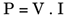Where:

V = Voltage (Joules/Coulomb or Volts)

I = Current (Coulombs/Sec or Amperes)

P = Power (Joules/Sec or Watts)

Now let's look at dissipation in Complementary Metal Oxide Semiconductor (CMOS) devices. CMOS technology is the best choice for low-power designs because of its insignificant static power dissipation. However, simply selecting CMOS technology should not be considered as the only method for reducing power in ASIC/SOC devices.

#### Sources of Power Dissipation in CMOS Devices

Since most of today's designs are based on CMOS technology, the first step toward power reduction is to understand the sources of power dissipation in such devices. Power consumption sources in digital CMOS circuits are divided into three main categories:

• Static power dissipation

• Short-circuit power dissipation

• Dynamic power dissipation

Figure 5.2 shows a CMOS inverter with these three sources of power consumption.

##### Figure 5.2. CMOS Power-Dissipation Parameters in an Inverter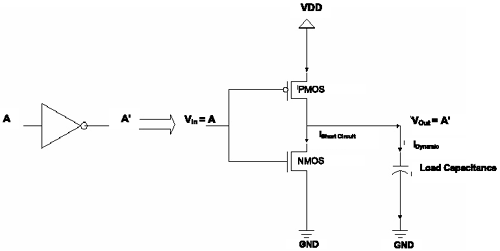Equation 5.2 illustrates the relationship between these three parameters.

Equation 5.2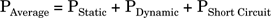CMOS devices have very low-static power dissipation and most of the energy in them is used to charge and discharge load capacitances. Figure 5.3 shows an example of charging and discharging the load capacitance in a CMOS inverter.

##### Figure 5.3. CMOS Inverter in Two Binary States (a) Charging the Load Capacitance; (b) Discharging the Load Capacitance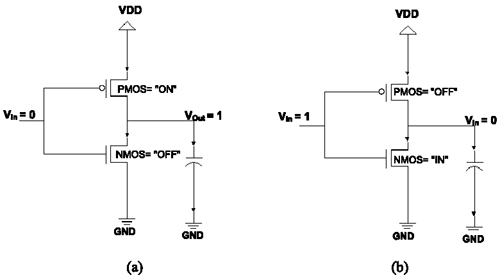As shown in Figure 5.3, the load capacitance in CMOS circuits is charged when positive voltage flows through PMOS transistor from V DD to represent logic 1. Similarly, the load capacitance is discharged through NMOS transistor to represent logic 0.

By comparison, the short-circuit and static powers are usually of smaller magnitude than the dynamic power, and they can be ignored. Therefore, dynamic power is the principal source of power dissipation in CMOS devices. The following sections explain each of these power dissipation sources in detail.

#### Static Power Dissipation

Static power dissipation occurs when the logic-gate output is stable; thus it is frequency independent. Equation 5.3 represents the static power components .

Equation 5.3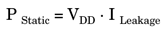Leakage current is caused by subthreshold-transistor operations and is determined by device technology. This type of current is responsible for power dissipation when a CMOS device is inactive and its value is insignificant (less than 1 percent) when the device is active. Therefore, the large amount of leakage current, or static power, accordingly is an indication of a serious design problem, such as static inputs that do not turn a gate on or off properly.

Static power dissipation in CMOS devices is usually negligible, because the amount of leakage current can be decreased significantly by choosing appropriate device technologies.

#### Short-Circuit Power Dissipation

As shown in Figure 5.3, short-circuit power dissipation occurs when current flows from power supply (V DD ) to ground (GND) during switching. The value of short-circuit dissipation depends on the amount of short-circuit current flowing to GND and it accounts for almost 10 percent of CMOS power consumption. Equation 5.4 represents the short-circuit power dissipation.

Equation 5.4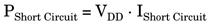Short-circuit current decreases when a large capacitive load is seen by the output of a gate and is at its maximum value when there is no capacitive load.

#### Dynamic Power Dissipation

Dynamic power is the dominant source of power dissipation in CMOS devices and accounts for approximately 90 percent of overall CMOS power consumption. It occurs during the switching of logic gates, and as a result, this type of power dissipation is frequency dependent. Dynamic power is therefore the average power required to perform all the switching events across the circuit. Equation 5.5 defines various parameters of dynamic power dissipation.

Equation 5.5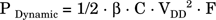Where:

b = Switching Activity per Node

C = Switched Capacitance

F = Frequency (switching events per second)

V DD = Supply Voltage

From Equation 5.5, it is evident that dynamic power can be reduced by lowering the supply voltage, switched capacitance, switching activity per node, or frequency of signal transitions from 0 to 1 or vice versa. It is also apparent from Equation 5.5 that the most effective and simple way of reducing dynamic power dissipation is by lowering the supply voltage (if the option of choosing lower voltage is available for a device). This is due to the squared effect of V DD . The other three terms in Equation 5.5 influence the overall power dissipation linearly.

The switching activity ( b ) determines the amount of switching that occurs in each node. Lowering this parameter decreases the useless transitions. b can be estimated statistically or captured from simulation traces (for example in Verilog, a .vcd file).

Frequency represents the switching events per second. Since dynamic power is frequency dependent, frequency reduction is a key concept in power optimization. Clocks are the major contributors to the frequency component of Equation 5.5. However, other signals such as bus interconnect signals contribute to high-frequency activity and should be lowered to optimize power.

Switched capacitance (C) can be either estimated based upon statistical models or measured from an actual layout database. Switched capacitance can be lowered by using shorter interconnect wires and smaller devices.Top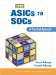From ASICs to SOCs: A Practical Approach
ISBN: 0130338575
EAN: 2147483647
Year: 2003
Pages: 61

Similar book on Amazon

flylib.com © 2008-2017.
If you may any questions please contact us: flylib@qtcs.net